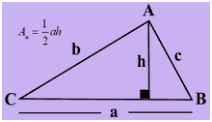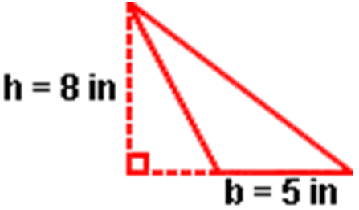# Area of a Triangle Formula

Triangle usually comprises of three edges and three vertices. Classified into three different types of triangles i.e. Equilateral, Isosceles and Scalene – each triangle has its own unique features and properties. If all three angles of a triangle are equal, the triangle is called Equilateral. A Isosceles triangle has two equal angles. A Scalene triangle is one in which all three angles are different. A right triangle has one right angle. In Geometry, two triangles are congruent if they are of the same shape and size.Area of a Triangle Formula is, $\frac{1}{2}$ah
Where,
a is the base of the triangle.
h is the height of the triangle.

### Solved Examples

Question 1: Find the area of an obtuse triangle with a base of 5 inches and a height of 8 inches.Solution:
Given,
b = 5 in
h = 8 in
Area of a triangle
= $\frac{1}{2}$ bh
= $\frac{1}{2}$ * 5 * 8 in2
= $\frac{40}{2}$ in2
= 20 in2
 More topics in Area of a Triangle Formula Area of Equilateral Triangle Formula

#### Practise This Question

A Man moves on a straight horizontal road with a block of 2kg in his hand. If he covers a distance of 20m with constant velocity of 1m/s. Find work done by man on block during motion.